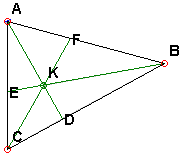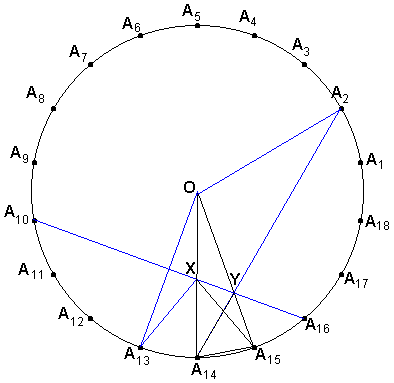# Trigonometric Form of Ceva's TheoremCeva's theorem provides a unifying concept for several apparently unrelated results. The theorem states that, in $\Delta ABC,$ three Cevians $AD,$ $BE,$ and $CF$ are concurrent iff the following identity holds:

$\displaystyle \frac{AF}{FB} \cdot \frac{BD}{DC} \cdot \frac{CE}{EA} = 1.$

The theorem has a less known trigonometric form

$\displaystyle \frac{\mbox{sin}(\angle ABE)}{\mbox{sin}(\angle CBE)} \cdot \frac{\mbox{sin}(\angle BCF)}{\mbox{sin}(\angle ACF)} \cdot \frac{\mbox{sin}(\angle CAD)}{\mbox{sin}(\angle BAD)} = 1,$

or

$\mbox{sin}(\angle ABE) \cdot \mbox{sin}(\angle BCF) \cdot \mbox{sin}(\angle CAD) = \mbox{sin}(\angle CBE) \cdot \mbox{sin}(\angle ACF) \cdot \mbox{sin}(\angle BAD).$

The latter may serve as a source of great many trigonometric identities - some obvious, some much less so. Some unexpected identities can be obtained by placing the six points $A,$ $B,$ $C$ at the vertices of a regular polygon. If the Cevians are extended to intersect the circumscribed circle, they become three diagonals (or sides) of the regular polygon. We are of course concerned with the case where a regular polygon has three concurrent diagonals.

For example, the original configuration in the applet below, suggests the following identity:

$\mbox{sin}(20^{\circ})\cdot \mbox{sin}(50^{\circ})\cdot \mbox{sin}(70^{\circ}) = \mbox{sin}(30^{\circ})\cdot \mbox{sin}(30^{\circ})\cdot \mbox{sin}(80^{\circ}),$

which can be easily verified. Play with the applet to find more such identities. The number of sides of the polygon, can be modified by clicking on the number - originally $18$ - in the lower left corner of the applet.

### This applet requires Sun's Java VM 2 which your browser may perceive as a popup. Which it is not. If you want to see the applet work, visit Sun's website at https://www.java.com/en/download/index.jsp, download and install Java VM and enjoy the applet.The applet also lends itself to discovery of problems of a different kind. Return to the $18-\mbox{gon}.$ You may observe that the configuration now is reminiscent of a very popular problem. Namely, in an isosceles $\Delta ABC,$ angle $B$ equals $20^{\circ}.$ (The same triangle appears in a different configuration inside regular $18-\mbox{gon}.$) Two lines $AD$ and $BE$ are drawn such that $\angle CAD = 60^{\circ},$ whereas $\angle ACE = 50^{\circ}.$ Find $\angle ADE.$ (Check a more extensive discussion of this problem and a relevant solution.)

From the diagram it is immediate that the answer is $30^{\circ}.$

In a similar vein consider another problem. In an isosceles ΔABC, $\angle ABC = 80^{\circ}.$ A point $M$ is selected so that $\angle MAC = 30^{\circ}$ and $\angle MCA = 10^{\circ}.$ Find $\angle BMC.$

Finally, see what you can make of the diagram below:### References

1. D. Gale, Tracking the Automatic Ant, Springer-Verlag, 1998
2. V. V. Prasolov, Essays On Numbers And Figures, AMS, 2000### Proof of Ceva's Trigonometric Identity$\displaystyle \frac{AF}{FB} \cdot \frac{BD}{DC} \cdot \frac{CE}{EA} = 1$

is equivalent to

$\displaystyle \frac{\mbox{Area}(\Delta AFK)}{\mbox{Area}(\Delta BFK)} \cdot \frac{\mbox{Area}(\Delta BKD)}{\mbox{Area}(\Delta CKD)} \cdot \frac{\mbox{Area}(\Delta CKE)}{\mbox{Area}(\Delta AKE)} = 1$

This is because triangles $AFK$ and $BFK$ have the same altitude from the vertex $K$ and similarly for other two pairs of triangles. On the other hand, $\mbox{Area}(\Delta AFK) = AF\cdot AK\cdot \mbox{sin}(\angle BAD)/2$, etc. which leads to

$\displaystyle \frac{\mbox{sin}(\angle ABE)}{\mbox{sin}(\angle CBE)} \cdot \frac{\mbox{sin}(\angle BCF)}{\mbox{sin}(\angle ACF)} \cdot \frac{\mbox{sin}(\angle CAD)}{\mbox{sin}(\angle BAD)} = \frac{FB}{AF} \cdot \frac{DC}{BD} \cdot \frac{EA}{CE} = 1,$

and the theorem follows.### ProblemIn an isosceles $\Delta ABC,$ $\angle ABC = 80^{\circ}.$ A point $M$ is selected so that $\angle MAC = 30^{\circ}$ and $\angle MCA = 10^{\circ}.$ Find $\angle BMC.$

Now, it's obvious that $\angle BMC = 70^{\circ}.$The following solution was found by S. T. Thompson, Tacoma, Washington (see Honsberger, pp 16-18).

With the change of notations, in $\Delta A_{14}OA_{15},$ two lines $A_{15}X$ and $A_{14}Y$ are drawn such that $\angle A_{14}A_{15}X = 60^{\circ}$ and $\angle A_{15}A_{14}Y = 50^{\circ}.$ The question in the above configuration is to determine $\angle A_{15}XY.$

Draw a circle with center $O$ and radius $OA_{14}.$ The chord $A_{14}A_{15}$ subtends a $20^{\circ}$ arc, so that $A_{14}A_{15}$ is a side of the regular $18-\mbox{gon}$ inscribed into that circle. I numbered the vertices of that $18-\mbox{gon}$ as shown in the diagram above.

Two observations are important for the proof:

1. $A_{14}A_{2}$ passes through $Y.$
2. $A_{10}A_{16}$ passes through both $X$ and $Y.$

Indeed, $A_{10}A_{16} = A_{14}A_{2},$ as chords subtending equal arcs. Furthermore, they are symmetric with respect to radius $OA_{15}.$ Therefore, they intersect on that radius. In the isosceles triangle $OA_{14}A_{2},$ the angle at $O$ is obviously equal $120^{\circ}.$ Therefore, $\angle OA_{14}A_{2} = 30^{\circ}.$ We see that $A_{14}A_{2}$ passes through $Y.$

Further, $A_{13}$ is the middle of the arc $A_{10}A_{16}.$ Therefore, $A_{10}A_{16} \perp OA_{13}.$

Let's for the moment denote the point of intersection of $A_{10}A_{16}$ with $OA_{14}$ as $X'.$ Since every point on $A_{10}A_{16}$ is equidistant from $O$ and $A_{13},$ so is $X':$ $OX' = X'A_{13}.$ In the isosceles triangle $OXA_{13},$ $\angle OA_{13}X = \angle A_{13}OX = 20^{\circ}.$ Therefore, $X' = X,$ which proves the second of the two observations.

Now, as we've seen, in the isosceles $OXA_{13},$ $A_{10}A_{16}$ is the height to side $OA_{13}.$ It's then bisects $\angle OXA_{13},$ which implies $\angle OXA_{10} = 70^{\circ}.$ But then also $\angle A_{14}XA_{16} = 70^{\circ}.$ On the other hand, $\angle A_{14}XA_{15} = 180^{\circ} - \angle OA_{14}A_{15} - \angle XA_{15}A_{14}.$ $\angle A_{14}XA_{15} = 180^{\circ} - 80^{\circ} - 60^{\circ} = 40^{\circ}.$ Finally, $\angle A_{15}XY = \angle A_{14}XA_{16} - \angle A_{14}XA_{15} = 70^{\circ} - 40^{\circ} = 30^{\circ}.$

### References

1. R. Honsberger, Mathematical Gems, II, MAA, 197671057804